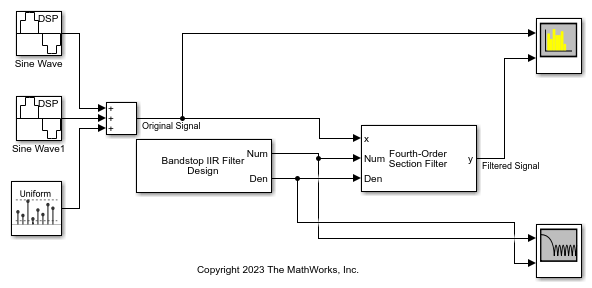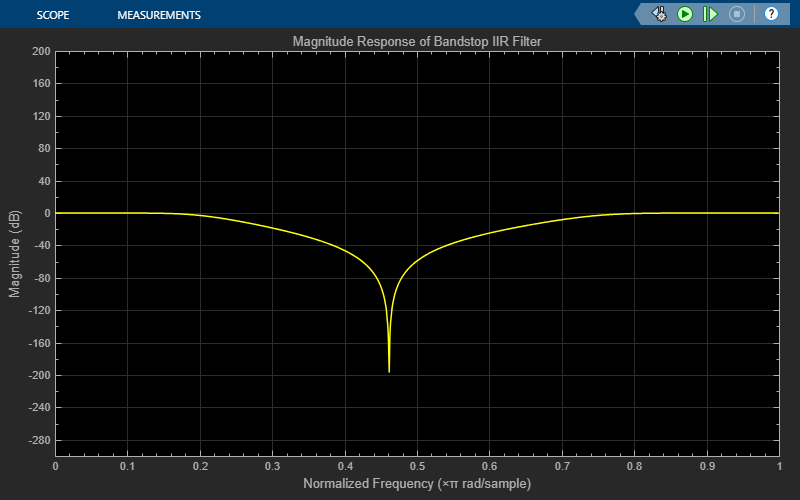# Tunable Bandstop IIR Filter

Design IIR bandstop filter with tunable filter specifications

Since R2023a

•Libraries:
DSP System Toolbox / Filtering / Filter Sources

## Description

Use the Tunable Bandstop IIR Filter block to design an IIR bandstop filter using the Butterworth, Chebyshev Type I and Chebyshev Type II design methods. You can tune the filter design specifications such as the filter order, 3-dB cutoff frequencies, passband ripple, and stopband attenuation while the simulation is running. When the values of these parameters change, the block redesigns the filter and outputs the numerator and denominator coefficients in the fourth-order section form.

## Examples

expand all

Filter a noisy sinusoidal signal using the Fourth-Order Section Filter block. The numerator and denominator coefficients of the fourth-order section filter are obtained using the Tunable Bandstop IIR Filter block. You can tune the frequency specifications of this filter during simulation.

Open and Run the Model

The input signal is a sum of two sine waves with the frequencies of 200 Hz and 400 Hz. The sample rate is 1000 Hz and the number of samples in each frame is 1024. Add zero-mean white Gaussian noise with a variance of 1e-4 to the sum of the sine waves.

The Tunable Bandstop IIR Filter block designs a sixth order bandpass IIR filter with the first and second 3-dB cutoff frequencies at 0.2rad/sample and 0.75rad/sample, respectively. Visualize the frequency response of the filter using Filter Visualizer.Filter the noisy sinusoidal signal using the Fourth-Order Section Filter block. Visualize the original sinusoidal signal and the filtered signal using the Spectrum Analyzer. The first tone is attenuated as it falls in the stopband region of the filter while the second tone remains unaffected as it falls in the passband region of the filter.Tuning Frequency Specification of FOS Filter

During simulation, you can tune the frequency specifications of the FOS filter by tuning the frequency parameters in the Tunable Bandstop IIR Filter block. The filter response updates accordingly.

Change the first 3-dB cutoff frequency to 0.5rad/sample in the Tunable Bandstop IIR Filter block. The first tone of the sinusoidal signal now falls in the passband region and is therefore unttenuated.## Ports

### Input

expand all

Specify the filter order as an even positive integer.

#### Dependency

To enable this port, select the Specify filter order from input port parameter.

Data Types: single | double | int8 | int16 | int32 | int64 | uint8 | uint16 | uint32 | uint64

Specify the first 3-dB cutoff frequency Fc1 of the filter in normalized frequency units as a nonnegative scalar less than or equal to the second 3-dB cutoff frequency Fc2.

#### Dependency

To enable this port, select the Specify first 3-dB cutoff frequency from input port parameter.

Data Types: single | double

Specify the second 3-dB cutoff frequency of the filter in normalized frequency units as a positive scalar greater than or equal to Fc1 and less than or equal to 1.

#### Dependency

To enable this port, select the Specify second 3-dB cutoff frequency from input port parameter.

Data Types: single | double

Specify the passband ripple of the Chebyshev Type I filter as a nonnegative scalar in dB.

#### Dependency

To enable this port:

• Set the Design method parameter to Chebyshev Type I.

• Select the Specify the passband ripple from input port parameter.

Data Types: single | double

Specify the stopband attenuation of the Chebyshev Type II filter as a nonnegative scalar in dB.

#### Dependency

To enable this port:

• Set the Design method parameter to Chebyshev Type II.

• Select the Specify the stopband attenuation from input port parameter.

Data Types: single | double

### Output

expand all

Numerator coefficients b, returned as a P-by-5 matrix (fourth-order section form), where P is the number of filter sections.

$b=\left[\begin{array}{ccccc}{b}_{01}& {b}_{11}& {b}_{21}& {b}_{31}& {b}_{41}\\ {b}_{02}& {b}_{12}& {b}_{22}& {b}_{32}& {b}_{42}\\ ⋮& ⋮& ⋮& \ddots & ⋮\\ {b}_{0P}& {b}_{1P}& {b}_{2P}& {b}_{3P}& {b}_{4P}\end{array}\right]$

This equation represents the fourth-order section filter in the transfer function form.

$H\left(z\right)=\prod _{k=1}^{P}{H}_{k}\left(z\right)=\prod _{k=1}^{P}\frac{{b}_{0k}+{b}_{1k}{z}^{-1}+{b}_{2k}{z}^{-2}+{b}_{3k}{z}^{-3}+{b}_{4k}{z}^{-4}}{{a}_{0k}+{a}_{1k}{z}^{-1}+{a}_{2k}{z}^{-2}+{a}_{3k}{z}^{-3}+{a}_{4k}{z}^{-4}}$

where,

• a –– Denominator coefficients matrix. For more details on how to specify this matrix, see the Den output port.

• k –– Row index.

The maximum number of filter sections equals ceil(Nmax/4), where Nmax is the value of the Filter maximum order (must be even) parameter.

When the actual filter order N is less than the maximum filter order Nmax, the last ceil(Nmax/4) − ceil(N/4) sections are trivial with coefficients [b0, b1, b2, b3, b4] = [1, 0, 0, 0, 0].

The data type of this port depends on the value of the Output data type parameter.

Data Types: single | double

Denominator coefficients a, returned as a P-by-5 matrix (fourth-order section form), where P is the number of filter sections.

$a=\left[\begin{array}{ccccc}{a}_{01}& {a}_{11}& {a}_{21}& {a}_{31}& {a}_{41}\\ {a}_{02}& {a}_{12}& {a}_{22}& {a}_{32}& {a}_{42}\\ ⋮& ⋮& ⋮& \ddots & ⋮\\ {a}_{0P}& {a}_{1P}& {a}_{2P}& {a}_{3P}& {a}_{4P}\end{array}\right]$

This equation represents the fourth-order section filter in the transfer function form.

$H\left(z\right)=\prod _{k=1}^{P}{H}_{k}\left(z\right)=\prod _{k=1}^{P}\frac{{b}_{0k}+{b}_{1k}{z}^{-1}+{b}_{2k}{z}^{-2}+{b}_{3k}{z}^{-3}+{b}_{4k}{z}^{-4}}{{a}_{0k}+{a}_{1k}{z}^{-1}+{a}_{2k}{z}^{-2}+{a}_{3k}{z}^{-3}+{a}_{4k}{z}^{-4}}$

where,

• b –– Numerator coefficients matrix. For more details on how to specify this matrix, see the Num output port.

• k –– Row index.

The maximum number of filter sections equals ceil(Nmax/4), where Nmax is the value of the Filter maximum order (must be even) parameter.

The leading denominator coefficient a0 is always 1.

When the actual filter order N is less than the maximum filter order Nmax, the last ceil(Nmax/4) − ceil(N/4) sections are trivial with coefficients [a0, a1, a2, a3, a4] = [1, 0, 0, 0, 0].

The data type of this port depends on the value of the Output data type parameter.

Data Types: single | double

## Parameters

expand all

Specify the filter design method as:

• Butterworth

• Chebyshev Type I

• Chebyshev Type II

Select this parameter to specify the filter order from the input port N. When you clear this parameter, you can specify the filter order in the block dialog box using the Filter order (must be even) parameter.

Specify the filter order as an even positive integer less than or equal to the value of the Filter maximum order (must be even) parameter.

Tunable: Yes

#### Dependency

To enable this parameter, clear the Specify filter order from input port parameter.

Specify the maximum order of the filter as an even positive integer. The value you specify in the Filter order (must be even) parameter must be less than or equal to the value you specify in the Filter maximum order (must be even) parameter.

Select this parameter to specify the first 3-dB filter cutoff frequency from the input port Fc1. When you clear this parameter, you can specify the first 3-dB cutoff frequency in the block dialog box using the First 3-dB cutoff frequency parameter.

Specify the first 3-dB cutoff frequency of the filter in normalized frequency units as a positive scalar less than or equal to the second 3-dB cutoff frequency.

Tunable: Yes

#### Dependency

To enable this parameter, clear the Specify first 3-dB cutoff frequency from input port parameter.

Select this parameter to specify the second 3-dB filter cutoff frequency from the input port Fc2. When you clear this parameter, you can specify the second 3-dB cut-off frequency in the block dialog box using the Second 3-dB cutoff frequency parameter.

Specify the second 3-dB cutoff frequency of the filter in normalized frequency units as a positive scalar greater than or equal to the first 3-dB cutoff frequency and less than or equal to 1.

Tunable: Yes

#### Dependency

To enable this parameter, clear the Specify second 3-dB cutoff frequency from input port parameter.

Select this parameter to specify passband ripple from the input port Ap. When you clear this parameter, you can specify the passband ripple in the block dialog box using the Passband ripple (dB) parameter.

#### Dependency

To enable this parameter, set the Design method parameter to Chebyshev Type I.

Specify the passband ripple of the Chebyshev Type I filter as a positive scalar in dB.

Tunable: Yes

#### Dependency

To enable this parameter:

• Set the Design method parameter to Chebyshev Type I.

• Clear the Specify the passband ripple from input port parameter.

Select this parameter to specify stopband attenuation from the input port Ast. When you clear this parameter, you can specify the stopband attenuation in the block dialog box using the Stopband attenuation (dB) parameter.

#### Dependency

To enable this parameter, set the Design method parameter to Chebyshev Type II.

Specify the stopband attenuation of the Chebyshev Type II filter as a positive scalar in dB.

Tunable: Yes

#### Dependency

To enable this parameter:

• Set the Design method parameter to Chebyshev Type II.

• Clear the Specify the stopband attenuation from input port parameter.

Specify the sample time as -1 (inherited), Inf (constant sample time), or a positive scalar. This parameter indicates when during the simulation the block produces the output and updates its internal state. For more details, see What Is Sample Time? (Simulink).

Specify the data type of the filter coefficients that the block outputs through the Num and Den ports as double or single.

Specify the type of simulation to run as one of the following:

• Code generation –– Simulate model using generated C code. The first time you run a simulation, Simulink® generates C code for the block. The C code is reused for subsequent simulations, as long as the model does not change. This option requires additional startup time but provides faster simulation speed than Interpreted execution.

• Interpreted execution –– Simulate model using the MATLAB®  interpreter. This option shortens startup time but has slower simulation speed than Code generation.

## Block Characteristics

 Data Types double | integer | single Direct Feedthrough no Multidimensional Signals no Variable-Size Signals no Zero-Crossing Detection no

 Orfanidis, Sophocles J. Introduction to Signal Processing. Prentice Hall, 1996.